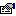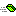## TripleDESCryptoServiceProvider

Provides cryptographic services using the TripleDES cryptographic service provider.

#### Remarks

This example shows a very simple method of encrypting then decrypting a String value.

`Private Sub Main()    ' Create a new TripleDESCryptoServiceProvider key.    Dim Key As New TripleDESCryptoServiceProvider    '' Encrypt a string to a byte array.    Dim Buffer() As Byte    Buffer = Encrypt("This is some plaintext!", Key)    '' Decrypt the byte array back to a string.    Dim PlainText As String    PlainText = Decrypt(Buffer, Key)    '' Display the plaintext value to the console.    Console.WriteLine PlainText    Console.ReadKeyEnd Sub 'Main'' Encrypt the string.Private Function Encrypt(ByVal PlainText As String, ByVal Key As SymmetricAlgorithm) As Byte()    Dim Buffer() As Byte    Buffer = StrConv(PlainText, vbFromUnicode)    Dim Encryptor As ICryptoTransform    Set Encryptor = Key.CreateEncryptor    Encrypt = Encryptor.TransformFinalBlock(Buffer, 0, cArray.GetLength(Buffer))End Function'' Decrypt the byte array.Public Function Decrypt(ByRef CypherText() As Byte, ByVal Key As SymmetricAlgorithm) As String    Dim Decryptor As ICryptoTransform    Set Decryptor = Key.CreateDecryptor        Dim Buffer() As Byte    Buffer = Decryptor.TransformFinalBlock(CypherText, 0, cArray.GetLength(CypherText))    Decrypt = StrConv(Buffer, vbUnicode)End Function`

### Public:

#### Properties:

NameDescriptionBlockSize (get)Returns the block size, in bits, used by this algorithm.BlockSize (let)Sets the block size, in bits, used by this algorithm.FeedbackSize (get)Returns the Feedback Size in bits.FeedbackSize (let)Sets the Feedback size in bits.IV (get)Returns the Initialization Vector.IV (let)Sets the Initialization Vector to be used.Key (get)Returns the current secret key.Key (let)Sets the secret key.KeySize (get)Returns the key size in bits.KeySize (let)Sets the key size in bits.LegalBlockSizes (get)Returns a list of legal block sizes.LegalKeySizes (get)Returns a list of valid key sizes supported by this algorithm.Mode (get)Returns the Cipher mode this algorithm will use.Mode (let)Sets the Cipher mode this algorithm will use.Padding (get)Returns the padding mode being used.Padding (let)Sets the padding mode to be used.

#### Methods:

NameDescriptionClearClears the Key and IV arrays.CreateDecryptorReturns a new cipher used to decrypt data.CreateEncryptorReturns a new cipher used to encrypt data.EqualsThis function determines if the value passed in is the same as the current object instance. Meaning, are the Value and this object the same object in memory.GenerateIVGenerates a random IV array to use.GenerateKeyGenerates a random key to be used.GetHashCodeReturns a psuedo-unique number used to help identify this object in memory. The current method is to return the value obtained from ObjPtr. If a different method needs to be impelmented then change the method here in this function.ToStringReturns a string representation of this object instance. The default method simply returns the application name and class name in which this class resides.ValidKeySizeTests if a specific bit length is valid for a key.